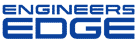# Re: loads on flange bolt patterns

[ Home ][ Forum Archive ] [ Active Engineering Forum ]

Posted By<" ">Neil Moran on March 14, 2002 at 15:49:12:

In Reply to: loads on flange bolt patterns posted byTim Lexen on January 30, 2002 at 12:56:00:

Hi,
Just found this forum out of the blue, so I am not sure if you still need an answer to this question.
The problem is not statically indeterminate -

For the `moment` on the bolts you need to determine the total `Z` of the bolt group. Assuming that the crane pivots about one of the bolts and taking the distance from this bolt to each of the other bolts as say d1, d2 ... dn (where dn is the furthest bolt) the total `Z` of the group is -

Z = (d1^2 + d2^2 + ... dn^2) / dn

You know what the total `moment` of the crane is, therefore -

Bolt load (max) i.e. the furthest bolt from assumed pivot bolt = Mmt / Z

The direct force on each bolt is just the total load divided by the number of bolts.

Hope this helps,
Neil Moran

Subject: Re: Re: loads on flange bolt patterns